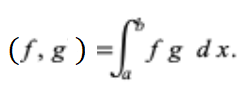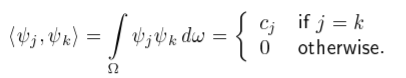# Orthogonal Functions & Orthonormal

Contents:

## What is orthogonality?

Orthogonality involves right angles. For example, two triangle side lengths at right angles to each other or two independent random variables. The two definition sound unrelated, but they are actually analogous in a mathematical sense:

Two independent random variables X and Y are related by the formula:
Var (X + Y) = Var(X) + Var(Y)
which is analogous to side lengths a and b, related to the hypotenuse in the Pythagorean theorem by √(a2 + b2) .

## Orthogonal Functions

Orthogonal functions are two functions with an inner product of zero.

Orthogonal functions are particularly useful for finding solutions to partial differential equations like Schrodinger’s equation and Maxwell’s equations.

## Orthogonal Functions and Inner Product Example

The inner product of two real-valued functions f and g on the closed interval [a, b] is given by the following definite integral:If the number given by the formula is zero, then the functions are orthogonal. You might see slightly different versions of this formula, but the underlying math is the same. For example, the following formula is specifically set up to deal with a steel rod heat conduction problem (Adomatis, n.d.):As well as the different function notation, note that the parentheses have been replaced by angled brackets < >. The angled brackets represent the inner product of two functions. It’s technically correct to use them, but I’m leaving them out here so that the following example is accessible to calculus students without any background in set theory or linear algebra.

## Example

Example question: Show that the functions f(x) = 1 + x and g(x) = x – x2 are orthogonal on the interval [-2, 2] by calculating the inner product.

Solution:

Step 1: Set up the definite integral for the inner product:Step 2: Solve the definite integral. I used a calculator (integral calculator) to get zero as a solution.

## Exception

Although the usual definition states that the inner product has to be zero in order for a function to be orthogonal, some functions are (perhaps strangely) orthogonal with themselves. For example, f(x) = cos (nx) is an orthogonal function over the closed interval [-π,π]. When the same function is evaluated using the inner product, it’s orthogonal if the inner product isn’t zero.

## Orthogonal Series

The general orthogonal series is represented by:All of the following series are special cases of these equations (WSU, 2020):

The theory of orthogonal series developed in mathematical physics as a result of solutions of problems via the Fourier method. Orthogonal series is one way to solve linear integral equations with symmetric kernels. The theory led to the conception of Hilbert space (Lyusternik & ‘Skii (1965).

## Orthonormal Functions

Orthonormal functions are orthogonal and normalized. They are often used to find approximations for other difficult to compute functions. You can construct any well behaved function f using a complete set of orthonormal functions (Errede, 2008):

f(x) = a0u0 + a1u1 + a2u2 + a3u3 + …

A “well behaved” function on an interval a ≥ x ≥ b is univariate, finite-valued and continuous (at least piecewise continuous).

The idea of creating new functions from a linear combination of orthogonal functions is similar to constructing a three-dimensional vector by combining the vectors (Levitus, 2020):

• v1 = {(1,0,0)}
• v1 = {(0,1,0)}
• v1 = {(0,0,1)}

## Properties of Orthogonal Functions

Orthonormal functions are normalized and mutually orthogonal; They are orthogonal functions with the property that the inner product ofn with itself is 1. Orthonormal functions are always linearly independent, which means that the maximum number of them in general n-dimensional space is equal to n. This set of linearly-independent, mutually orthogonal, normalized functions in n-dimensional space is called an orthonormal basis function (Bishop, 1993).

## Example: Orthonormal Functions and Representation of Signals

A set of signals can be represented by a set of orthonormal basis functions; All possible
linear combinations are called a signal space (which is a function-space coordinate system). The coordinate axes in this space are the orthonormal functions u<1sub>1(t), u(t), …, un(t). The major benefit of performing this series expansion is that once this space has been created, any signal can be represented as a point in space, which means that you can use “usual” vector theory.

## References

 Kjos-Hanssen, B. (2019). Statistics for Calculus Students. Retrieved April 28, 2021 from: https://dspace.lib.hawaii.edu/bitstream/10790/4572/s4cs.pdf

Admonatis, R. (n.d.). Chapter 8. Orthogonal Function Sequences. Retrieved February 13, 2020 from: https://user.eng.umd.edu/~adomaiti/ench453-648/ch8.pdf
Bishop, D. (1993). Group Theory and Chemistry. Dover Publications.
Bringhurst, R. (2002). The Elements of Typographical Style.
Orthogonal Functions. Retrieved February 13, 2020 from: http://dslavsk.sites.luc.edu/courses/phys301/classnotes/morthogonality.pdf
Errede, S. (2008). Supplemental Handout #1: Orthogonal Functions & Expansions. Retrieved November 20, 2020 from: https://courses.physics.illinois.edu/phys435/sp2010/Lecture_Notes/P435_Supp_HO_01.pdf
Gram-Schmidt. 21. Orthonormal Representation of Signals. Retrieved November 20, 2020 from: http://charleslee.yolasite.com/resources/elec321/lect_gram_schmidt.pdf
Levitus, M. (2020). Orthogonal Expansions. licensed by CC BY-NC-SA 3.0. Retrieved November 20, 2020 from: https://chem.libretexts.org/Bookshelves/Physical_and_Theoretical_Chemistry_Textbook_Maps/Book%3A_Mathematical_Methods_in_Chemistry_(Levitus)/07%3A_Fourier_Series/7.03%3A_Orthogonal_Expansions
L. Lyusternik & A.’Skii (Eds.)(1965). Chapter IV. Orthogonal Series and Orthogonal Systems. Pergamon. Retrieved November 18, 2020 from: http://www.sciencedirect.com/science/article/pii/B9781483166889500077
WSU Research Foundation. Introduction to Orthogonal Series. Retrieved November 18, 2020 from: http://www.sci.wsu.edu/math/faculty/barnes/fourier.htm

CITE THIS AS:
Stephanie Glen. "Orthogonal Functions & Orthonormal" From StatisticsHowTo.com: Elementary Statistics for the rest of us! https://www.statisticshowto.com/orthogonal-functions/